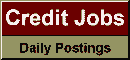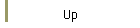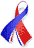DefaultRisk.com the web's biggest credit risk modeling resource.Export citation to:- HTML- Text (plain)- BibTeX- RIS- ReDIF

Distribution-Invariant Risk Measures, Entropy, and Large Deviations

by Stefan Weber of Cornell University

December 4, 2006

Abstract: The simulation of distributions of financial positions is an important issue for financial institutions. If risk measures are evaluated for a simulated distribution instead of the model-implied distribution, errors of risk measurements needs to be analyzed. For distribution-invariant risk measures which are continuous on compacts we employ the theory of large deviations to study the probability of large errors. If the approximate risk measurements are based on the empirical distribution of independent samples, the rate function equals the minimal relative entropy under a risk measure constraint. For shortfall risk and average value at risk (AVaR) we solve this minimization problem explicitly.

Keywords: Risk measures, average value at risk, shortfall risk, Monte Carlo, large deviation principle, Sanov's theorem, relative entropy.

Published in: Journal of Applied Probability, Vol. 44, No. 1, (March 2007), pp. 16-40.

Books Referenced in this paper:  (what is this?)